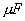Monday 13th July 2020
 CBSE Guess > Papers > Question Papers > Class XII > 2005 > Physics > Outside Delhi Set-II PHYSICS 2005 (Set II—Outside Delhi)
Except for the following questions all the remaining questions have been asked in Set I..  Q. 1. What should be the length of a dipole antenna for a carrier wave of frequency 6 x 108 Hz? 1 Q. 2. Show graphically how the stopping potential for a given photosensitive surfa- ce varies with the frequency of the incident radiation. 1 Q. 3. In a series LCR circuit, the voltage across an inductor, capacitor and resistor are 20 V, 20 V and 40 V respectively. What is the phase difference between the applied voltage and the current in the circuit? 1 Q. 4. Under what condition does an electron moving through a magnetic field experience maximum force? 1 Q. 5. How does the coulomb force between two point charges depend upon the dielectric constant of the intervening medium? 1 Q. 10. Establish a relation between current and drift velocity. 1 Q. 11. A charge q is Placed at the centre of the line joining two equal charges Q. Show that the system of three charges will be in equilibrium if q = - Q/4. 2 Or A 5capacitor is charged by a 100 V supply. The supply is then disconnected and the charged capacitor is connected to another uncharged 3capacitor. How much electrostatic energy of the first capacitor is lost in the process of attaining the steady situation? Q. 13. The potential difference across the terminals of a battery of emf 12 V and internal resistance 2 ohm drops to 10 V when it is connected to a silver voltameter. Calculate the silver deposited at the cathode in half an hour. Relative atomic mass of silver is 108. 3 Q. 15. Define mutual inductance and give its S. I . unit. Derive an express on for the mutual inductance of two long coaxial solenoids of same length wound one over the other. 3 Q. 17. A double convex lens made of glass of refractive index 1.6 has its both surfaces of equal radii of curvature of 30 cm each. An object of 5 cm height is placed at a distance of 12.5 cm from the lens. Find the position, nature and size of the image. 3 Physics 2005 Question Papers Class XII Delhi Outside Delhi Compartment Delhi Compartment Outside Delhi Set I Set I Set I Set I Set II Set II Set II Set II Set III Set III Set III CBSE 2005 Question Papers Class XII